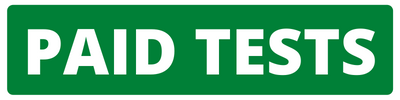CAT | Cognizant Logical Questions

## Cognizant Logical QuestionsJobs By Batch
Jobs By Location
Jobs By Degree
Jobs By Branch
IT Jobs
Internships
Govt. Jobs• ##### Original Questions of IT Companies| All Topics### Quiz Begins Here

Q #1
:

#### Cognizant Logical Question

Select the right option from the given alternatives. 64 : 56 :: 81 :
+

As difference between 64 and 56 is 8, Similarly 81-8=73.

Q #2
:

#### Cognizant Logical Question

�A� driven 10 km towards East and turns to the right hand driven 3 km. Then he drives towards West (turning at his right) 3 km. He then turns to his left and drives 2 km. finally he turns to his right and travels 7 km. how far is he from his starting point and in which direction would he be?
+

A driven 10 km towards East and turns to south driven 3 km. Then he drives towards West 3 km. He then turns to south and drives 2 km. finally he turns to west and travels 7 km and reaches 5km towards South.

Q #3
:

#### Cognizant Logical Question

From the given anagrams select the odd one out.
+

Difference between 1st and and 2nd letter and between 2nd and 3rd letter is same except MQT where difference between first two letters is 4 and between last two letters is 3.

Q #4
:

#### Cognizant Logical Question

Given signs signify something and on the basis, assume the given statements to be true and find which of the two conclusions I & II is/are definitely true.

A+B means A is greater than equal to B
A-B means A is equal to B
A \$ means A is less than B
A*B means A is equal to B
A/B means A is greater than B

Statements: D*G, G-H, H/J

Conclusions: I. D ≠ H II. G/J

+

D=G=H>J
Therefore, only option II, G>J is correct.

Q #5
:

#### Cognizant Logical Question

Given signs signify something and on that basis, assume the given statements to be true and find which of the two conclusions I and II is/are definitely true. "�" denotes "greater than" "+" denotes "equal to" "-" denotes "not equal than" "/" denotes "smaller than" "%" denotes "not less than" "*" denotes "not greater than" A*B implies
+

A<=B , Therefore none is true.